# KARNAUGH MAPPING

Why learn about Karnaugh maps? The Karnaugh map, like Boolean algebra, is a simplification tool applicable to digital logic. See the “Toxic waste incinerator” in the Boolean algebra chapter for an example of Boolean simplification of digital logic. The Karnaugh Map will simplify logic faster and more easily in most cases.

Boolean simplification is actually faster than the Karnaugh map for a task involving two or fewer Boolean variables. It is still quite usable at three variables, but a bit slower. At four input variables, Boolean algebra becomes tedious. Karnaugh maps are both faster and easier. Karnaugh maps work well for up to six input variables, are usable for up to eight variables. For more than six to eight variables, simplification should be by CAD (computer automated design).In theory any of the three methods will work. However, as a practical matter, the above guidelines work well. We would not normally resort to computer automation to simplify a three input logic block. We could sooner solve the problem with pencil and paper. However, if we had seven of these problems to solve, say for a BCD (Binary Coded Decimal) to seven segment decoder, we might want to automate the process. A BCD to seven segment decoder generates the logic signals to drive a seven segment LED (light emitting diode) display.

Examples of computer automated design languages for simplification of logic are PALASM, ABEL, CUPL, Verilog, and VHDL. These programs accept a hardware descriptor language input file which is based on Boolean equations and produce an output file describing a reduced (or simplified) Boolean solution. We will not require such tools in this chapter. Let’s move on to Venn diagrams as an introduction to Karnaugh maps.

## Venn diagrams and sets

Mathematicians use Venn diagrams to show the logical relationships of sets (collections of objects) to one another. Perhaps you have already seen Venn diagrams in your algebra or other mathematics studies. If you have, you may remember overlapping circles and the union and intersection of sets. We will review the overlapping circles of the Venn diagram. We will adopt the terms OR and AND instead of union and intersection since that is the terminology used in digital electronics.

The Venn diagram bridges the Boolean algebra from a previous chapter to the Karnaugh Map. We will relate what you already know about Boolean algebra to Venn diagrams, then transition to Karnaugh maps.

A set is a collection of objects out of a universe as shown below. The members of the set are the objects contained within the set. The members of the set usually have something in common; though, this is not a requirement. Out of the universe of real numbers, for example, the set of all positive integers {1,2,3…} is a set. The set {3,4,5} is an example of a smaller set, or subset of the set of all positive integers. Another example is the set of all males out of the universe of college students. Can you think of some more example of sets?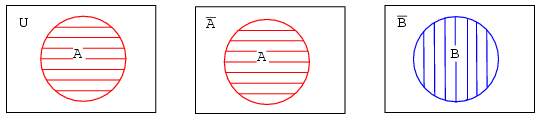Above left, we have a Venn diagram showing the set A in the circle within the universe U, the rectangular area. If everything inside the circle is A, then anything outside of the circle is not A. Thus, above center, we label the rectangular area outside of the circle A as A-not instead of U. We show B and B-not in a similar manner.

What happens if both A and B are contained within the same universe? We show four possibilities.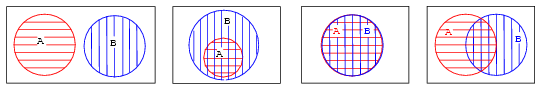Let’s take a closer look at each of the the four possibilities as shown above.The first example shows that set A and set B have nothing in common according to the Venn diagram. There is no overlap between the A and B circular hatched regions. For example, suppose that sets A and B contain the following members:

set A = {1,2,3,4}
set B = {5,6,7,8}

None of the members of set A are contained within set B, nor are any of the members of B contained within A. Thus, there is no overlap of the circles.In the second example in the above Venn diagram, Set A is totally contained within set B How can we explain this situation? Suppose that sets A and B contain the following members:

set A = {1,2} set B = {1,2,3,4,5,6,7,8}

All members of set A are also members of set B. Therefore, set A is a subset of Set B. Since all members of set A are members of set B, set A is drawn fully within the boundary of set B.

There is a fifth case, not shown, with the four examples. Hint: it is similar to the last (fourth) example. Draw a Venn diagram for this fifth case.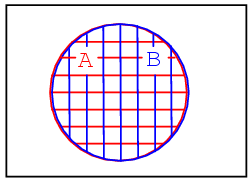The third example above shows perfect overlap between set A and set B. It looks like both sets contain the same identical members. Suppose that sets A and B contain the following:

set A = {1,2,3,4} set B = {1,2,3,4}

Therefore,

Set A = Set B

Sets And B are identically equal because they both have the same identical members. The A and B regions within the corresponding Venn diagram above overlap completely. If there is any doubt about what the above patterns represent, refer to any figure above or below to be sure of what the circular regions looked like before they were overlapped.The fourth example above shows that there is something in common between set A and set B in the overlapping region. For example, we arbitrarily select the following sets to illustrate our point:

set A = {1,2,3,4}
set B = {3,4,5,6}

Set A and Set B both have the elements 3 and 4 in common These elements are the reason for the overlap in the center common to A and B. We need to take a closer look at this situation

## Boolean Relationships on Venn Diagrams

The fourth example has A partially overlapping B. Though, we will first look at the whole of all hatched area below, then later only the overlapping region. Let’s assign some Boolean expressions to the regions above as shown below.
Below left there is a red horizontal hatched area for A. There is a blue vertical hatched area for B.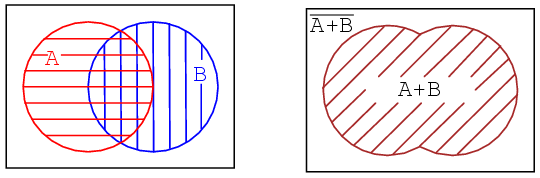If we look at the whole area of both, regardless of the hatch style, the sum total of all hatched areas, we get the illustration above right which corresponds to the inclusive OR function of A, B. The Boolean expression is A+B. This is shown by the 45o hatched area. Anything outside of the hatched area corresponds to (A+B)-not as shown aboe. Let’s move on to next part of the fourth example

The other way of looking at a Venn diagram with overlapping circles is to look at just the part common to both A and B, the double hatched area below left. The Boolean expression for this common area corresponding to the AND function is AB as shown below right. Note that everything outside of double hatched AB is AB-not.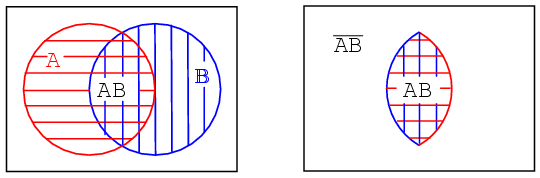Note that some of the members of A, above, are members of (AB)’. Some of the members of B are members of (AB)’. But, none of the members of (AB)’ are within the doubly hatched area AB.We have repeated the second example above left. Your fifth example, which you previously sketched, is provided above right for comparison. Later we will find the occasional element, or group of elements, totally contained within another group in a Karnaugh map.

Next, we show the development of a Boolean expression involving a complemented variable below.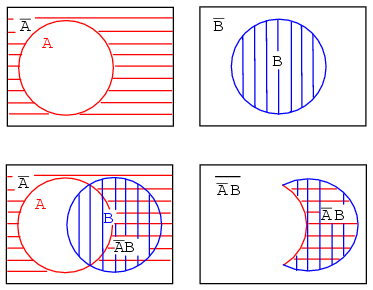Example: (above)

Show a Venn diagram for A’B (A-not AND B).

Solution:

Starting above top left we have red horizontal shaded A’ (A-not), then, top right, B. Next, lower left, we form the AND function A’B by overlapping the two previous regions. Most people would use this as the answer to the example posed. However, only the double hatched A’B is shown far right for clarity. The expression A’B is the region where both A’ and B overlap. The clear region outside of A’B is (A’B)’, which was not part of the posed example.

Let’s try something similar with the Boolean OR function.

Example:

Find B’+A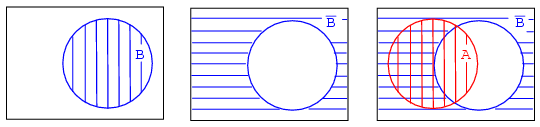Solution:

Above right we start out with B which is complemented to B’. Finally we overlay A on top of B’. Since we are interested in forming the OR function, we will be looking for all hatched area regardless of hatch style. Thus, A+B’ is all hatched area above right. It is shown as a single hatch region below left for clarity.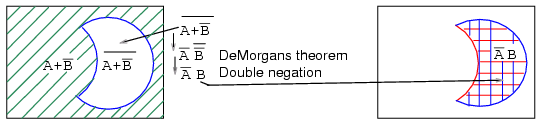Example:

Find (A+B’)’

Solution:

The green 45o A+B’ hatched area was the result of the previous example. Moving on to a to,(A+B’)’ ,the present example, above left, let us find the complement of A+B’, which is the white clear area above left corresponding to (A+B’)’. Note that we have repeated, at right, the AB’ double hatched result from a previous example for comparison to our result. The regions corresponding to (A+B’)’ and AB’ above left and right respectively are identical. This can be proven with DeMorgan’s theorem and double negation.

This brings up a point. Venn diagrams don’t actually prove anything. Boolean algebra is needed for formal proofs. However, Venn diagrams can be used for verification and visualization. We have verified and visualized DeMorgan’s theorem with a Venn diagram.

Example:

What does the Boolean expression A’+B’ look like on a Venn Diagram?Solution: above figure

Start out with red horizontal hatched A’ and blue vertical hatched B’ above. Superimpose the diagrams as shown. We can still see the A’ red horizontal hatch superimposed on the other hatch. It also fills in what usedto be part of the B (B-true) circle, but only that part of the B open circle not common to the A open circle. If we only look at the B’ blue vertical hatch, it fills that part of the open A circle not common to B. Any region with any hatch at all, regardless of type, corresponds to A’+B’. That is, everything but the open white space in the center.

Example:

What does the Boolean expression (A’+B’)’ look like on a Venn Diagram?

Solution: above figure, lower left

Looking at the white open space in the center, it is everything NOT in the previous solution of A’+B’, which is (A’+B’)’.

Example:

Show that (A’+B’) = AB

Solution: below figure, lower left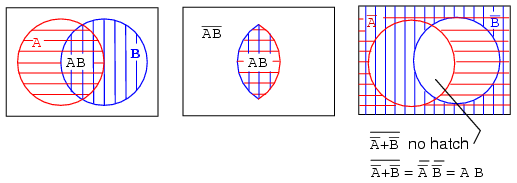Related

COMMENT songs

1. Guru

Karnaugh map
The Karnaugh map (K-map for short), Maurice Karnaugh’s 1953 refinement of Edward Veitch’s 1952 Veitch diagram, is a method to simplify Boolean algebra expressions …
In a Karnaugh map the boolean variables are transferred (generally from a truth table) and ordered according to the principles of Gray code in which only one …
The K-Map method may theoretically be applied for the simplification of any boolean expression regardless of its number of variables, but …
History – Properties – Example – Race hazards
http://en.wikipedia.org/wiki/Karnaugh_map

Now that we have developed the Karnaugh map with the aid of Venn diagrams, let’s put it to use. Karnaugh maps reduce logic functions more quickly and …
First is relay ladder logic, then logic gates, a truth table, a Karnaugh map, and a Boolean equation. The point is that any of these are equivalent. Two …
Note that this same output α is found in the Karnaugh map at the A=0, B=0 cell address, upper left corner of K-map where the A=0 row and B=0 column …

Digital Logic – Karnaugh Maps
Karnaugh Maps are used for many small design problems. It’s true that many larger designs are done using computer implementations of different algorithms. However …
In this section we’ll examine some Karnaugh Maps for three and four variables. As we use them be particularly tuned in to how they are really being …
A Karnaugh Map is a grid-like representation of a truth table. It is really just another way of presenting a truth table, but the mode of presentation …
http://www.facstaff.bucknell.edu/mastascu/elessonshtml/

A Karnaugh map (K-map) is a pictorial method used to minimize Boolean expressions without having to use Boolean algebra theorems and equation manipulations.
Using a K-map, expressions with two to four variables are easily minimized. Expressions with five to six variables are more difficult but achievable, and expressions with seven or more variables are extremely difficult (if not impossible) to minimize using a K-map. …
http://whatis.techtarget.com/definition/0,,sid9_gci804449,00.html

Karnaugh Map Minimizer
Karnaugh Map Minimizer is free (GPL) software for minimizing boolean functions using the graphic method of Karnaugh maps. I …
http://k-map.sourceforge.net/

Karnaugh Map
Karnaugh Map simplification program.
If you do so, the truth table will be updated real-time to reflect the Karnaugh map. The program constantly updates the screen, drawing the largest circle around the group of adjacent bits. The …
The price of kmap 4 is \$15.00. You may purchase this with Visa or Master Card. Verification of card takes 1 minute and download takes an additional minute and a half on a 28.8 Kbps modem. kmap445 …
http://www.puz.com/sw/karnaugh/

Karnaugh Maps
The Karnaugh map provides a simple and straight-forward method of minimising boolean expressions. With the Karnaugh map Boolean expressions having up to four …
A Karnaugh map provides a pictorial method of grouping together expressions with common factors and therefore eliminating unwanted variables. The …
The diagram below illustrates the correspondence between the Karnaugh map and the truth table for the general case of a two variable problem. …
http://www.ee.surrey.ac.uk/Projects/Labview/

KMAP Home
The KMAP Web site provides health and medical policy information to beneficiaries and providers. Our vision is to connect Kansans with …
https://www.kmap-state-ks.us/ – Cached – Similar

Karnaugh Minimizer | Easy Karnaugh maps for everyone
Draws 2 – 8 variable Karnaugh Maps; Quine Mc Cluskey minimization tool allow you to handle 9-23 variables. Convert boolean formula to VHDL or Verilog …
http://karnaugh.shuriksoft.com

Karnaugh Map Explorer – HOME – Electrical Engineering Department …
Click on the buttons in the Truth Table or in the Karnaugh Map to change the value. Mouse over minterm components of the function F to see a representation …
http://courseware.ee.calpoly.edu/~rsandige/KarnaughExplorer.html

2. pjp

k map

3. Polprav

Hello from Russia!
Can I quote a post in your blog with the link to you?